# sklearn.inspection.PartialDependenceDisplay¶

class sklearn.inspection.PartialDependenceDisplay(pd_results, *, features, feature_names, target_idx, deciles, kind='average', subsample=1000, random_state=None, is_categorical=None)[source]

Partial Dependence Plot (PDP).

This can also display individual partial dependencies which are often referred to as: Individual Condition Expectation (ICE).

It is recommended to use from_estimator to create a PartialDependenceDisplay. All parameters are stored as attributes.

Read more in Advanced Plotting With Partial Dependence and the User Guide.

New in version 0.22.

Parameters:
pd_resultslist of Bunch

Results of partial_dependence for features.

featureslist of (int,) or list of (int, int)

Indices of features for a given plot. A tuple of one integer will plot a partial dependence curve of one feature. A tuple of two integers will plot a two-way partial dependence curve as a contour plot.

feature_nameslist of str

Feature names corresponding to the indices in features.

target_idxint
• In a multiclass setting, specifies the class for which the PDPs should be computed. Note that for binary classification, the positive class (index 1) is always used.

• In a multioutput setting, specifies the task for which the PDPs should be computed.

Ignored in binary classification or classical regression settings.

decilesdict

Deciles for feature indices in features.

kind{‘average’, ‘individual’, ‘both’} or list of such str, default=’average’

Whether to plot the partial dependence averaged across all the samples in the dataset or one line per sample or both.

• kind='average' results in the traditional PD plot;

• kind='individual' results in the ICE plot;

• kind='both' results in plotting both the ICE and PD on the same plot.

A list of such strings can be provided to specify kind on a per-plot basis. The length of the list should be the same as the number of interaction requested in features.

Note

ICE (‘individual’ or ‘both’) is not a valid option for 2-ways interactions plot. As a result, an error will be raised. 2-ways interaction plots should always be configured to use the ‘average’ kind instead.

Note

The fast method='recursion' option is only available for kind='average' and sample_weights=None. Computing individual dependencies and doing weighted averages requires using the slower method='brute'.

New in version 0.24: Add kind parameter with 'average', 'individual', and 'both' options.

New in version 1.1: Add the possibility to pass a list of string specifying kind for each plot.

subsamplefloat, int or None, default=1000

Sampling for ICE curves when kind is ‘individual’ or ‘both’. If float, should be between 0.0 and 1.0 and represent the proportion of the dataset to be used to plot ICE curves. If int, represents the maximum absolute number of samples to use.

Note that the full dataset is still used to calculate partial dependence when kind='both'.

New in version 0.24.

random_stateint, RandomState instance or None, default=None

Controls the randomness of the selected samples when subsamples is not None. See Glossary for details.

New in version 0.24.

is_categoricallist of (bool,) or list of (bool, bool), default=None

Whether each target feature in features is categorical or not. The list should be same size as features. If None, all features are assumed to be continuous.

New in version 1.2.

Attributes:
bounding_ax_matplotlib Axes or None

If ax is an axes or None, the bounding_ax_ is the axes where the grid of partial dependence plots are drawn. If ax is a list of axes or a numpy array of axes, bounding_ax_ is None.

axes_ndarray of matplotlib Axes

If ax is an axes or None, axes_[i, j] is the axes on the i-th row and j-th column. If ax is a list of axes, axes_[i] is the i-th item in ax. Elements that are None correspond to a nonexisting axes in that position.

lines_ndarray of matplotlib Artists

If ax is an axes or None, lines_[i, j] is the partial dependence curve on the i-th row and j-th column. If ax is a list of axes, lines_[i] is the partial dependence curve corresponding to the i-th item in ax. Elements that are None correspond to a nonexisting axes or an axes that does not include a line plot.

deciles_vlines_ndarray of matplotlib LineCollection

If ax is an axes or None, vlines_[i, j] is the line collection representing the x axis deciles of the i-th row and j-th column. If ax is a list of axes, vlines_[i] corresponds to the i-th item in ax. Elements that are None correspond to a nonexisting axes or an axes that does not include a PDP plot.

New in version 0.23.

deciles_hlines_ndarray of matplotlib LineCollection

If ax is an axes or None, vlines_[i, j] is the line collection representing the y axis deciles of the i-th row and j-th column. If ax is a list of axes, vlines_[i] corresponds to the i-th item in ax. Elements that are None correspond to a nonexisting axes or an axes that does not include a 2-way plot.

New in version 0.23.

contours_ndarray of matplotlib Artists

If ax is an axes or None, contours_[i, j] is the partial dependence plot on the i-th row and j-th column. If ax is a list of axes, contours_[i] is the partial dependence plot corresponding to the i-th item in ax. Elements that are None correspond to a nonexisting axes or an axes that does not include a contour plot.

bars_ndarray of matplotlib Artists

If ax is an axes or None, bars_[i, j] is the partial dependence bar plot on the i-th row and j-th column (for a categorical feature). If ax is a list of axes, bars_[i] is the partial dependence bar plot corresponding to the i-th item in ax. Elements that are None correspond to a nonexisting axes or an axes that does not include a bar plot.

New in version 1.2.

heatmaps_ndarray of matplotlib Artists

If ax is an axes or None, heatmaps_[i, j] is the partial dependence heatmap on the i-th row and j-th column (for a pair of categorical features) . If ax is a list of axes, heatmaps_[i] is the partial dependence heatmap corresponding to the i-th item in ax. Elements that are None correspond to a nonexisting axes or an axes that does not include a heatmap.

New in version 1.2.

figure_matplotlib Figure

Figure containing partial dependence plots.

partial_dependence

Compute Partial Dependence values.

PartialDependenceDisplay.from_estimator

Plot Partial Dependence.

Examples

>>> import numpy as np
>>> import matplotlib.pyplot as plt
>>> from sklearn.datasets import make_friedman1
>>> from sklearn.inspection import PartialDependenceDisplay
>>> from sklearn.inspection import partial_dependence
>>> X, y = make_friedman1()
>>> features, feature_names = [(0,)], [f"Features #{i}" for i in range(X.shape)]
>>> deciles = {0: np.linspace(0, 1, num=5)}
>>> pd_results = partial_dependence(
...     clf, X, features=0, kind="average", grid_resolution=5)
>>> display = PartialDependenceDisplay(
...     [pd_results], features=features, feature_names=feature_names,
...     target_idx=0, deciles=deciles
... )
>>> display.plot(pdp_lim={1: (-1.38, 0.66)})
<...>
>>> plt.show()


Methods

 from_estimator(estimator, X, features, *[, ...]) Partial dependence (PD) and individual conditional expectation (ICE) plots. plot(*[, ax, n_cols, line_kw, ice_lines_kw, ...]) Plot partial dependence plots.
classmethod from_estimator(estimator, X, features, *, sample_weight=None, categorical_features=None, feature_names=None, target=None, response_method='auto', n_cols=3, grid_resolution=100, percentiles=(0.05, 0.95), method='auto', n_jobs=None, verbose=0, line_kw=None, ice_lines_kw=None, pd_line_kw=None, contour_kw=None, ax=None, kind='average', centered=False, subsample=1000, random_state=None)[source]

Partial dependence (PD) and individual conditional expectation (ICE) plots.

Partial dependence plots, individual conditional expectation plots or an overlay of both of them can be plotted by setting the kind parameter. The len(features) plots are arranged in a grid with n_cols columns. Two-way partial dependence plots are plotted as contour plots. The deciles of the feature values will be shown with tick marks on the x-axes for one-way plots, and on both axes for two-way plots.

Read more in the User Guide.

Note

PartialDependenceDisplay.from_estimator does not support using the same axes with multiple calls. To plot the partial dependence for multiple estimators, please pass the axes created by the first call to the second call:

>>> from sklearn.inspection import PartialDependenceDisplay
>>> from sklearn.datasets import make_friedman1
>>> from sklearn.linear_model import LinearRegression
>>> from sklearn.ensemble import RandomForestRegressor
>>> X, y = make_friedman1()
>>> est1 = LinearRegression().fit(X, y)
>>> est2 = RandomForestRegressor().fit(X, y)
>>> disp1 = PartialDependenceDisplay.from_estimator(est1, X,
...                                                 [1, 2])
>>> disp2 = PartialDependenceDisplay.from_estimator(est2, X, [1, 2],
...                                                 ax=disp1.axes_)


Warning

For GradientBoostingClassifier and GradientBoostingRegressor, the 'recursion' method (used by default) will not account for the init predictor of the boosting process. In practice, this will produce the same values as 'brute' up to a constant offset in the target response, provided that init is a constant estimator (which is the default). However, if init is not a constant estimator, the partial dependence values are incorrect for 'recursion' because the offset will be sample-dependent. It is preferable to use the 'brute' method. Note that this only applies to GradientBoostingClassifier and GradientBoostingRegressor, not to HistGradientBoostingClassifier and HistGradientBoostingRegressor.

New in version 1.0.

Parameters:
estimatorBaseEstimator

A fitted estimator object implementing predict, predict_proba, or decision_function. Multioutput-multiclass classifiers are not supported.

X{array-like, dataframe} of shape (n_samples, n_features)

X is used to generate a grid of values for the target features (where the partial dependence will be evaluated), and also to generate values for the complement features when the method is 'brute'.

featureslist of {int, str, pair of int, pair of str}

The target features for which to create the PDPs. If features[i] is an integer or a string, a one-way PDP is created; if features[i] is a tuple, a two-way PDP is created (only supported with kind='average'). Each tuple must be of size 2. If any entry is a string, then it must be in feature_names.

sample_weightarray-like of shape (n_samples,), default=None

Sample weights are used to calculate weighted means when averaging the model output. If None, then samples are equally weighted. If sample_weight is not None, then method will be set to 'brute'. Note that sample_weight is ignored for kind='individual'.

New in version 1.3.

categorical_featuresarray-like of shape (n_features,) or shape (n_categorical_features,), dtype={bool, int, str}, default=None

Indicates the categorical features.

• None: no feature will be considered categorical;

• boolean array-like: boolean mask of shape (n_features,) indicating which features are categorical. Thus, this array has the same shape has X.shape;

• integer or string array-like: integer indices or strings indicating categorical features.

New in version 1.2.

feature_namesarray-like of shape (n_features,), dtype=str, default=None

Name of each feature; feature_names[i] holds the name of the feature with index i. By default, the name of the feature corresponds to their numerical index for NumPy array and their column name for pandas dataframe.

targetint, default=None
• In a multiclass setting, specifies the class for which the PDPs should be computed. Note that for binary classification, the positive class (index 1) is always used.

• In a multioutput setting, specifies the task for which the PDPs should be computed.

Ignored in binary classification or classical regression settings.

response_method{‘auto’, ‘predict_proba’, ‘decision_function’}, default=’auto’

Specifies whether to use predict_proba or decision_function as the target response. For regressors this parameter is ignored and the response is always the output of predict. By default, predict_proba is tried first and we revert to decision_function if it doesn’t exist. If method is 'recursion', the response is always the output of decision_function.

n_colsint, default=3

The maximum number of columns in the grid plot. Only active when ax is a single axis or None.

grid_resolutionint, default=100

The number of equally spaced points on the axes of the plots, for each target feature.

percentilestuple of float, default=(0.05, 0.95)

The lower and upper percentile used to create the extreme values for the PDP axes. Must be in [0, 1].

methodstr, default=’auto’

The method used to calculate the averaged predictions:

Please see this note for differences between the 'brute' and 'recursion' method.

n_jobsint, default=None

The number of CPUs to use to compute the partial dependences. Computation is parallelized over features specified by the features parameter.

None means 1 unless in a joblib.parallel_backend context. -1 means using all processors. See Glossary for more details.

verboseint, default=0

Verbose output during PD computations.

line_kwdict, default=None

Dict with keywords passed to the matplotlib.pyplot.plot call. For one-way partial dependence plots. It can be used to define common properties for both ice_lines_kw and pdp_line_kw.

ice_lines_kwdict, default=None

Dictionary with keywords passed to the matplotlib.pyplot.plot call. For ICE lines in the one-way partial dependence plots. The key value pairs defined in ice_lines_kw takes priority over line_kw.

pd_line_kwdict, default=None

Dictionary with keywords passed to the matplotlib.pyplot.plot call. For partial dependence in one-way partial dependence plots. The key value pairs defined in pd_line_kw takes priority over line_kw.

contour_kwdict, default=None

Dict with keywords passed to the matplotlib.pyplot.contourf call. For two-way partial dependence plots.

axMatplotlib axes or array-like of Matplotlib axes, default=None
• If a single axis is passed in, it is treated as a bounding axes and a grid of partial dependence plots will be drawn within these bounds. The n_cols parameter controls the number of columns in the grid.

• If an array-like of axes are passed in, the partial dependence plots will be drawn directly into these axes.

• If None, a figure and a bounding axes is created and treated as the single axes case.

kind{‘average’, ‘individual’, ‘both’}, default=’average’

Whether to plot the partial dependence averaged across all the samples in the dataset or one line per sample or both.

• kind='average' results in the traditional PD plot;

• kind='individual' results in the ICE plot.

Note that the fast method='recursion' option is only available for kind='average' and sample_weights=None. Computing individual dependencies and doing weighted averages requires using the slower method='brute'.

centeredbool, default=False

If True, the ICE and PD lines will start at the origin of the y-axis. By default, no centering is done.

New in version 1.1.

subsamplefloat, int or None, default=1000

Sampling for ICE curves when kind is ‘individual’ or ‘both’. If float, should be between 0.0 and 1.0 and represent the proportion of the dataset to be used to plot ICE curves. If int, represents the absolute number samples to use.

Note that the full dataset is still used to calculate averaged partial dependence when kind='both'.

random_stateint, RandomState instance or None, default=None

Controls the randomness of the selected samples when subsamples is not None and kind is either 'both' or 'individual'. See Glossary for details.

Returns:
displayPartialDependenceDisplay

partial_dependence

Compute Partial Dependence values.

Examples

>>> import matplotlib.pyplot as plt
>>> from sklearn.datasets import make_friedman1
>>> from sklearn.inspection import PartialDependenceDisplay
>>> X, y = make_friedman1()
>>> PartialDependenceDisplay.from_estimator(clf, X, [0, (0, 1)])
<...>
>>> plt.show()

plot(*, ax=None, n_cols=3, line_kw=None, ice_lines_kw=None, pd_line_kw=None, contour_kw=None, bar_kw=None, heatmap_kw=None, pdp_lim=None, centered=False)[source]

Plot partial dependence plots.

Parameters:
axMatplotlib axes or array-like of Matplotlib axes, default=None
• If a single axis is passed in, it is treated as a bounding axes

and a grid of partial dependence plots will be drawn within these bounds. The n_cols parameter controls the number of columns in the grid.

• If an array-like of axes are passed in, the partial dependence

plots will be drawn directly into these axes.

• If None, a figure and a bounding axes is created and treated

as the single axes case.

n_colsint, default=3

The maximum number of columns in the grid plot. Only active when ax is a single axes or None.

line_kwdict, default=None

Dict with keywords passed to the matplotlib.pyplot.plot call. For one-way partial dependence plots.

ice_lines_kwdict, default=None

Dictionary with keywords passed to the matplotlib.pyplot.plot call. For ICE lines in the one-way partial dependence plots. The key value pairs defined in ice_lines_kw takes priority over line_kw.

New in version 1.0.

pd_line_kwdict, default=None

Dictionary with keywords passed to the matplotlib.pyplot.plot call. For partial dependence in one-way partial dependence plots. The key value pairs defined in pd_line_kw takes priority over line_kw.

New in version 1.0.

contour_kwdict, default=None

Dict with keywords passed to the matplotlib.pyplot.contourf call for two-way partial dependence plots.

bar_kwdict, default=None

Dict with keywords passed to the matplotlib.pyplot.bar call for one-way categorical partial dependence plots.

New in version 1.2.

heatmap_kwdict, default=None

Dict with keywords passed to the matplotlib.pyplot.imshow call for two-way categorical partial dependence plots.

New in version 1.2.

pdp_limdict, default=None

Global min and max average predictions, such that all plots will have the same scale and y limits. pdp_lim is the global min and max for single partial dependence curves. pdp_lim is the global min and max for two-way partial dependence curves. If None (default), the limit will be inferred from the global minimum and maximum of all predictions.

New in version 1.1.

centeredbool, default=False

If True, the ICE and PD lines will start at the origin of the y-axis. By default, no centering is done.

New in version 1.1.

Returns:
displayPartialDependenceDisplay

Returns a PartialDependenceDisplay object that contains the partial dependence plots.

## Examples using sklearn.inspection.PartialDependenceDisplay¶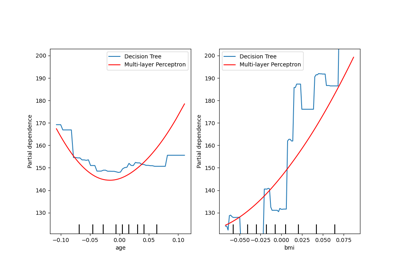## Examples using sklearn.inspection.PartialDependenceDisplay.from_estimator¶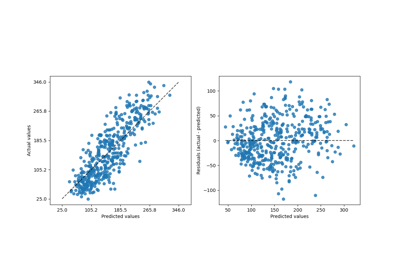Release Highlights for scikit-learn 1.2

Release Highlights for scikit-learn 1.2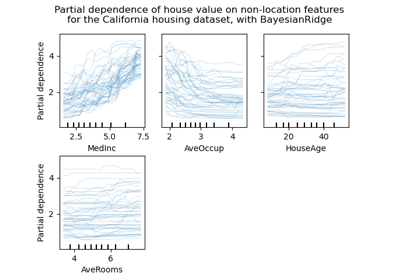Release Highlights for scikit-learn 0.24

Release Highlights for scikit-learn 0.24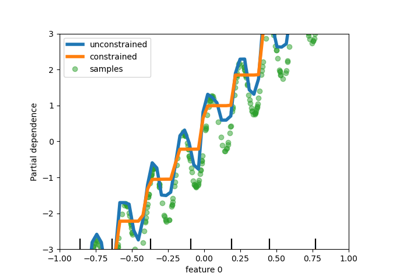Release Highlights for scikit-learn 0.23

Release Highlights for scikit-learn 0.23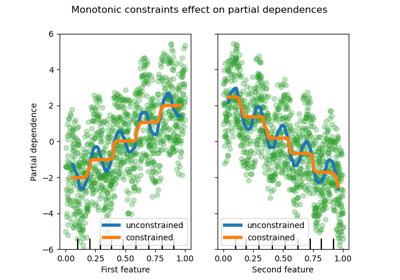Monotonic Constraints

Monotonic Constraints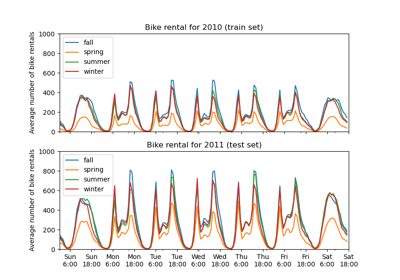Partial Dependence and Individual Conditional Expectation Plots

Partial Dependence and Individual Conditional Expectation Plots